# ASP 实现将数据导出到 Excel 文件中

【摘要】本文记录一下在 ASP 中将数据导入到 Excel 文件中的几个步骤，这里 excel 的格式是 ".xlsx" 而不是 ".xls"，这是有区别的，如果使用 ".xls" 来保存文件，那么使用 excel 打开的时候会报错。然而使用浏览器下载 ".xlsx" 的时候也会报错，而本文仅仅记录一下 ASP 将 excel 生成到 1.xlsx 在网站目录中，而不能在客户端浏览器中下载。

1. `'--如果原来的 EXCEL 文件存在的话则删除它`
2. `Set fs = server.CreateObject("scripting.filesystemobject")`
3. `filename = "江南大学计算机科学与技术2015级.xlsx"`
4. `file = "H:\ASP\XWY\" & filename`
5. `if fs.FileExists(file) then`
6. `   fs.DeleteFile(file)`
7. `end  if`
8. `set fs = Nothing`
9. `Set ExcelApp = CreateObject("Excel.Application")`
10. `ExcelApp.Application.Visible = True  `
11. `Set ExcelBook = ExcelApp.Workbooks.Add`
12. `ExcelBook.WorkSheets(1).cells(1,1).value = "学号"`
13. `ExcelBook.WorkSheets(1).cells(1,2).value = "姓名"`
14. `ExcelBook.WorkSheets(1).cells(1,3).value = "手机号码"`
15. `ExcelBook.WorkSheets(1).cells(2,1).value = "6151910036"`
16. `ExcelBook.WorkSheets(1).cells(2,2).value = "马富天"`
17. `ExcelBook.WorkSheets(1).cells(2,3).value = "17095248823"`
18. `ExcelBook.WorkSheets(1).cells(3,1).value = "6151910035"`
19. `ExcelBook.WorkSheets(1).cells(3,2).value = "吕海峰"`
20. `ExcelBook.WorkSheets(1).cells(3,3).value = "18806186012"`
21. `Excelbook.SaveAs file`
22. `ExcelApp.Application.Quit  `
23. `Set ExcelApp = Nothing`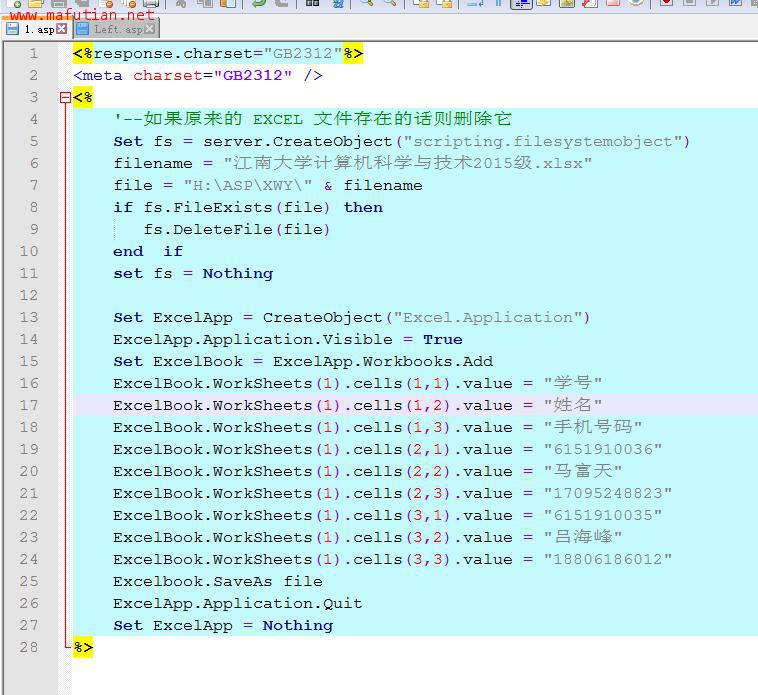Windows 下需要如下操作：

（也可以通过运行->DCOMCNFG来启动组件服务配置）

0

0

 评论审核未开启 回复后邮件通知我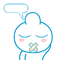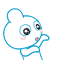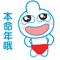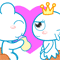• 浏览最多
• 评论最多